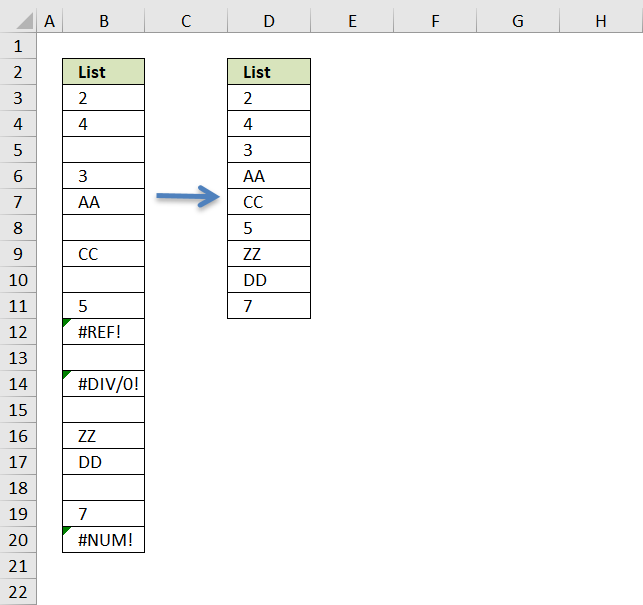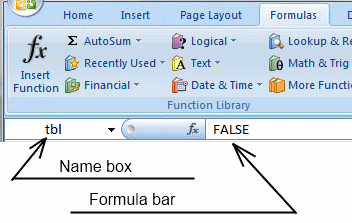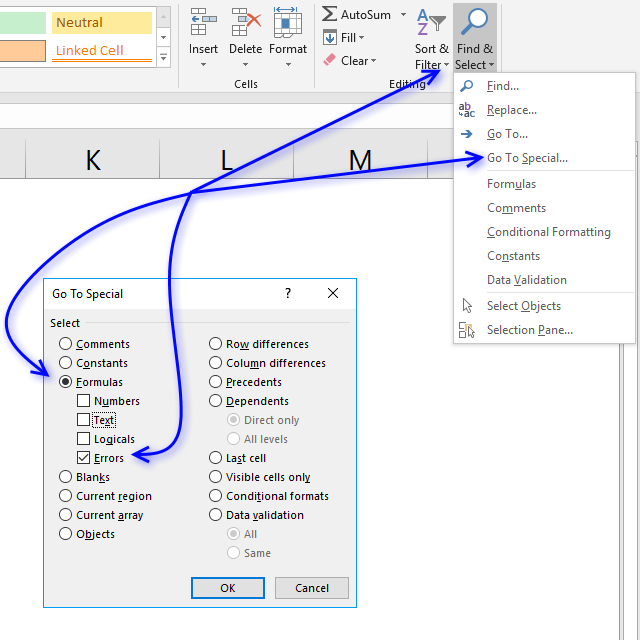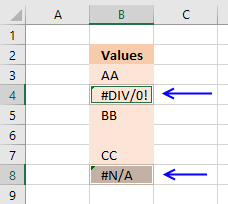Author: Oscar Cronquist Article last updated on March 14, 2022

## 1. Delete blanks and errors in a list

The formula deletes blank cells and cells with errors. It doesn't matter if the cells contain numbers or text, they all will be presented in a new column.### Array formula in cell D3:

=INDEX(\$B\$3:\$B\$20, SMALL(IF(ISBLANK(\$B\$3:\$B\$20)+ISERROR(\$B\$3:\$B\$20), "", MATCH(ROW(\$B\$3:\$B\$20),ROW(\$B\$3:\$B\$20))), ROWS(\$A\$1:A1)))

### How to create an array formula

1. Copy (Ctrl + c) and paste (Ctrl + v) array formula into formula bar. See picture below.
2. Press and hold Ctrl + Shift.
3. Press Enter once.
4. Release all keys.### How to copy array formula

1. Copy (Ctrl + c) cell D3
2. Paste (Ctrl + v) array formula on cell range D3:D11

### Explaining formula in cell D3

#### Step 1 - Identify blank cells

The ISBLANK function returns TRUE if cell is blank (empty) and FALSE if not.

ISBLANK(\$B\$3:\$B\$20)

becomes

ISBLANK({2; 4; 0; 3; "AA"; 0; "CC"; 0; 5; #REF!; 0; #DIV/0!; 0; "ZZ"; "DD"; 0; 7; #NUM!})

and returns

{FALSE; FALSE; TRUE; FALSE; FALSE; TRUE; FALSE; TRUE; FALSE; FALSE; TRUE; FALSE; TRUE; FALSE; FALSE; TRUE; FALSE; FALSE}

#### Step 2 - Identify errors

The ISERROR function returns TRUE if cell contains an error and FALSE if not.

ISERROR(\$B\$3:\$B\$20)

becomes

ISERROR({2; 4; 0; 3; "AA"; 0; "CC"; 0; 5; #REF!; 0; #DIV/0!; 0; "ZZ"; "DD"; 0; 7; #NUM!})

and returns

{FALSE; FALSE; FALSE; FALSE; FALSE; FALSE; FALSE; FALSE; FALSE; TRUE; FALSE; TRUE; FALSE; FALSE; FALSE; FALSE; FALSE; TRUE}

#### Step 3 - Add arrays

If at least one of the boolean values is TRUE then the result must be TRUE, addition is what we need to use.

 Boolean Boolean Multiply Add FALSE FALSE 0  (zero) 0 (zero) FALSE TRUE 0  (zero) 1 TRUE TRUE 1 2

ISBLANK(\$B\$3:\$B\$20)+ISERROR(\$B\$3:\$B\$20)

becomes

{FALSE; FALSE; TRUE; FALSE; FALSE; TRUE; FALSE; TRUE; FALSE; FALSE; TRUE; FALSE; TRUE; FALSE; FALSE; TRUE; FALSE; FALSE} + {FALSE; FALSE; FALSE; FALSE; FALSE; FALSE; FALSE; FALSE; FALSE; TRUE; FALSE; TRUE; FALSE; FALSE; FALSE; FALSE; FALSE; TRUE}

returns {0; 0; 1; 0; 0; 1; 0; 1; 0; 1; 1; 1; 1; 0; 0; 1; 0; 1}.

#### Step 4 - Convert array to row numbers

The IF function lets you use a logical expression to determine which value (argument) to return.

IF(ISBLANK(\$B\$3:\$B\$20)+ISERROR(\$B\$3:\$B\$20), "", MATCH(ROW(\$B\$3:\$B\$20),ROW(\$B\$3:\$B\$20)))

becomes

IF({0; 0; 1; 0; 0; 1; 0; 1; 0; 1; 1; 1; 1; 0; 0; 1; 0; 1}, "", {1;2;3;4;5;6;7;8;9;10;11;12;13;14;15;16;17;18})

and returns

{1;2;"";4;5;"";7;"";9;"";"";"";"";14;15;"";17;""}

#### Step 5 - Get k-th smallest row number

To be able to return a single value from the array we need to use the SMALL function to extract a single row number. The second argument in the SMALL function uses the ROWS function with an expanding cell reference to extract a new value in each cell.

SMALL(IF(ISBLANK(\$B\$3:\$B\$20)+ISERROR(\$B\$3:\$B\$20), "", MATCH(ROW(\$B\$3:\$B\$20),ROW(\$B\$3:\$B\$20))), ROWS(\$A\$1:A1))

becomes

SMALL({1;2;"";4;5;"";7;"";9;"";"";"";"";14;15;"";17;""}, ROWS(\$A\$1:A1))

becomes

SMALL({1;2;"";4;5;"";7;"";9;"";"";"";"";14;15;"";17;""}, 1)

and returns 1.

#### Step 6 - Return value based on row number

The INDEX function returns a value from a cell range based on a row and column number, our cell range is a single column so we need to only specify a row number in order to get the correct value.

INDEX(\$B\$3:\$B\$20, SMALL(IF(ISBLANK(\$B\$3:\$B\$20)+ISERROR(\$B\$3:\$B\$20), "", MATCH(ROW(\$B\$3:\$B\$20),ROW(\$B\$3:\$B\$20))), ROWS(\$A\$1:A1)))

becomes

INDEX(\$B\$3:\$B\$20, 1)

becomes

INDEX({2;4;0;3;"AA";0;"CC";0;5;#REF!;0;#DIV/0!;0;"ZZ";"DD";0;7;#NUM!}, 1)

and returns 2 in cell D3.

### Get excel *.xls

remove-blanks.xls

## 2. How to find errors in a worksheetExcel has great built-in features. The following one lets you search an entire worksheet for formulas that return an error.

Instructions:

1. Go to "Home" tab2. Press with left mouse button on "Find & Select"
3. Press with left mouse button on "Go to Special..."
4. Press with left mouse button on "Formulas"
5. Enable "Errors"6. Press with left mouse button on ok!

If any formula errors exist, they are now selected. The picture below demonstrates error cells being selected.